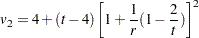Multivariate Inferences

Multivariate inference based on Wald tests can be done withimputed data sets. The approach is a generalization of the approach taken in the univariate case (Rubin 1987, p. 137; Schafer 1997, p. 113). Suppose that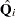and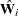are the point and covariance matrix estimates for a-dimensional parameter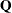(such as a multivariate mean) from the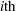imputed data set,= 1, 2, ...,. Then the combined point estimate forfrom the multiple imputation is the average of thecomplete-data estimates: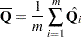Suppose that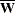is the within-imputation covariance matrix, which is the average of thecomplete-data estimates: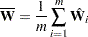And suppose that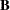is the between-imputation covariance matrix: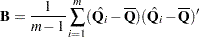Then the covariance matrix associated with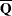is the total covariance matrix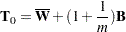The natural multivariate extension of thestatistic used in the univariate case is thestatistic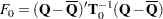with degrees of freedomand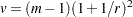where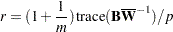is an average relative increase in variance due to nonresponse (Rubin 1987, p. 137; Schafer 1997, p. 114).

However, the reference distribution of the statistic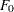is not easily derived. Especially for small, the between-imputation covariance matrixis unstable and does not have full rank for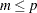(Schafer 1997, p. 113).

One solution is to make an additional assumption that the population between-imputation and within-imputation covariance matrices are proportional to each other (Schafer 1997, p. 113). This assumption implies that the fractions of missing information for all components ofare equal. Under this assumption, a more stable estimate of the total covariance matrix is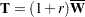With the total covariance matrix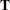, thestatistic (Rubin 1987, p. 137)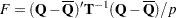has andistribution with degrees of freedomand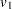, where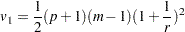For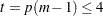, PROC MIANALYZE uses the degrees of freedomin the analysis. For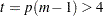, PROC MIANALYZE uses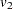, a better approximation of the degrees of freedom given by Li, Raghunathan, and Rubin (1991):